|

# 一进门玄关风水画怎么选?有品位更旺财的选择

玄关是一种挡门煞的存在，在家中有着实用价值以及风水价值，对于正大门的玄关来说我们需要注重其装饰的美观度，亦要注意其中的风水元素，玄关挂画是有品位的人家中的刚需，装饰画的选择能起到提升玄关品味的作用，还能够改善家中玄关的风水，那么究竟正对大门的玄关挂什么字画风水好呢?易从网推荐旺财玄关字画还得选择山水画!山水画和中国的风水紧密相连，山水画中的一山一树一草一木皆被风水赋予了特殊的含义，比如山主丁水旺财的，水在山水画是一个重要的元素，亦是灵魂所在，水为活，亦为财，不动的水为死水，财不通，所以这就是人们为何在选择山水画的时候都喜欢有瀑布的，飞湍不息的瀑布，意指自己的财运滔滔不绝，源源流淌，财源广进!

正对大门玄关挂画：

热销玄关风水画 李林宏新品山水画《福地安居图》作品来源：易从网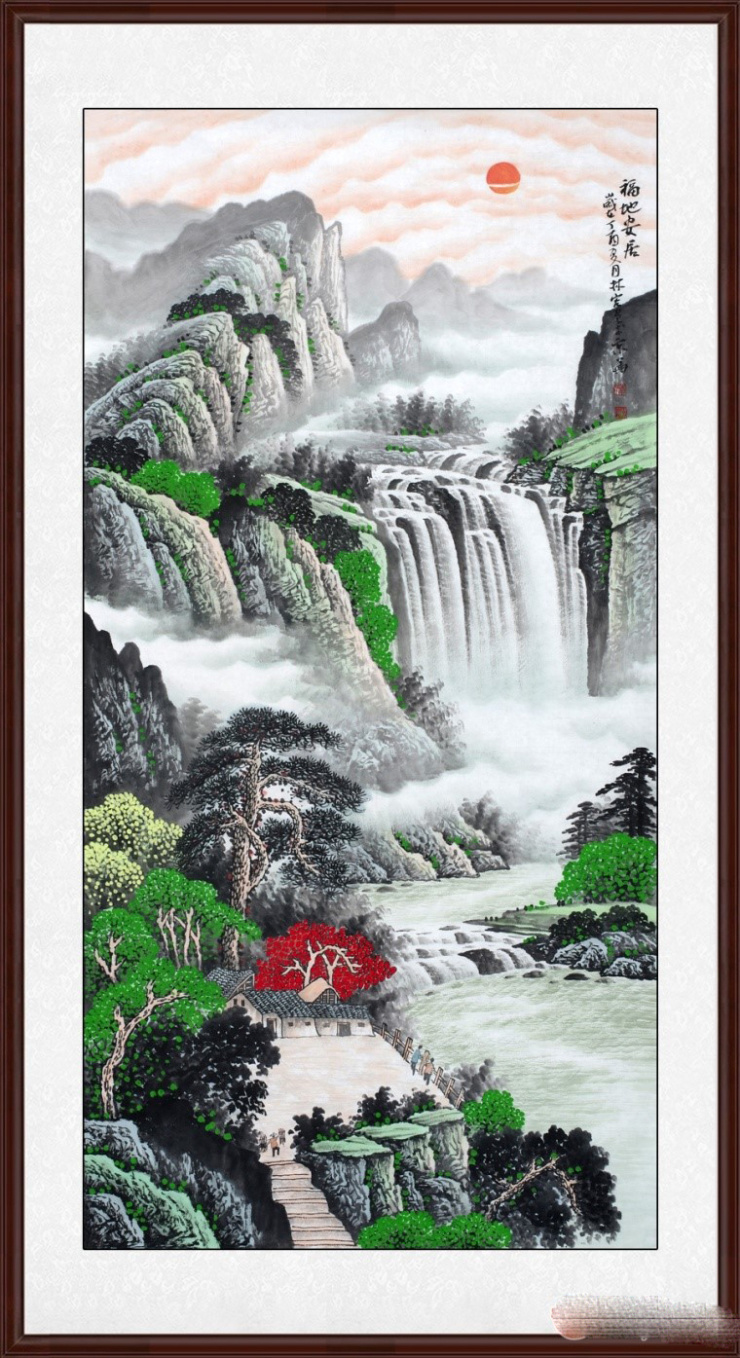因为玄关的空间限制，所以一般玄关挂画都是以竖幅山水画为主，李林宏老师的这幅《福地安居图》山水画就极为合适，先抛开其他的，就看其中的太阳就是一种浩然正气的存在，玄关挡煞旭日东升绝对是最佳的选择，给人一种正大光明的感觉，飞流的瀑布，开门见财运，满眼的春意盎然，无论是自己还是客人绝对都会有耳目一新的感觉!

潺潺流水云雾缠绕，有民宅静谧安然，气势磅礴的全景山水，峰峦层叠，植被丰茂，有草木微染秋色，其间居住的人家，得山水清汽，度悠哉人生。展现春天的独特意境，线条灵活多变，墨色晕染有致，墨色典雅，布局严谨，画面里山石险峻，草木葱茏，溪流清澈，房屋建于山脚下，凭栏而望，满眼美景，令人羡慕不已，挂在玄关更具艺术品味!

正对大门玄关挂画：

玄关招财画 李林宏旺财风水画佳作《紫气东来》作品来源：易从网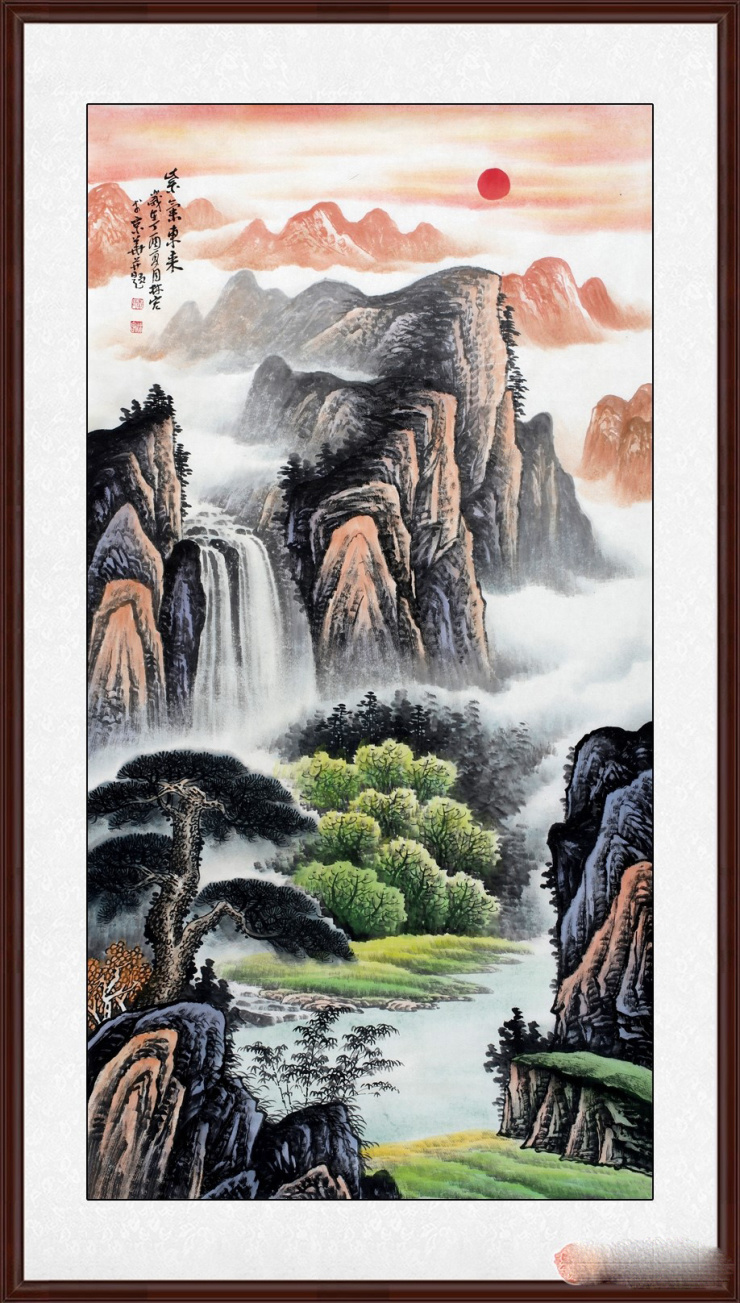天赐祥云。云海茫茫，烟霞云霁，表润泽肥沃，紫气东来;祥云天降瑞气千条吉祥伴随。千年古松，招财挡灾。古树参天，葱葱绿绿，表朝气勃勃，旺盛之气;具有招财进宝辟邪挡灾之意。迎门富贵竹，竹报平安，大富大贵，山延绵，即龙脉延绵不绝，或高或低，或耸或入，开腾飞跃，展现的是龙的气势滂沱。山厚实，展示的是龙脉的雄厚坚实;不管是做生意或者是事业上都会遇到靠山。水的部份代表财源,富水长流(源远流长取之不尽用之不竭)，流动的水如瀑布等更是川流不息的好兆头。流动的水汇聚成“聚宝盆”形状，寓意财源的聚集。这幅山水画挂于玄关招财迎贵，富贵有余!

正对大门玄关挂画：

小品斗方画 李林宏新作山水画《迎客松》作品来源：易从网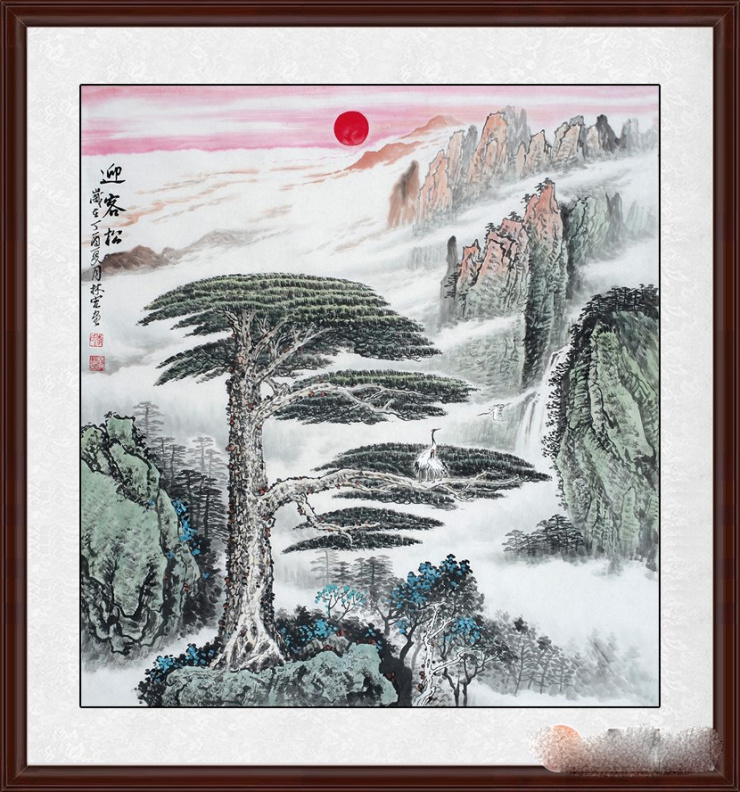君子之正气选择，黄山迎客松，桀骜不驯，英姿飒爽，谦恭有礼!

迎客松也是一种欢迎宾客到来之意，所以挂在家中极为合适，谦恭有礼，君子大方之气，这幅画将浩荡的天地之间氤氲之气跃入画卷，灵气，福气，财气将相随融通，丰泽绵绵。迎客松在风水学中，寓意指迎福招财。挂在家中遮灾避难，旺财运。中国人视松为吉祥物,松被视作“百木之长”,称作“木公”、“大夫”.松的特点是凌霜不凋、冬夏常青.苍松劲挺,饱含风霜而生机勃勃!因此,古人视松作长青之树,古代有长生不老松之说,人们赋予其延年益寿、长青不老的吉祥寓意.松也是吉祥的梦兆.松更普遍地是被视作祝颂、祈盼青春永驻、健康长寿的象征物。

松除了是一种长寿的象征外，也常常作为有志有节的代表和象征。松鹤延年则寓延年益寿或为志节清高之意。亦有称“松鹤同春”。此幅作品不仅适合挂在家里，更是祝寿礼品的首选!

正对大门玄关挂画：

斗方聚宝盆风水画 李林宏山水画《源远流长》作品来源：易从网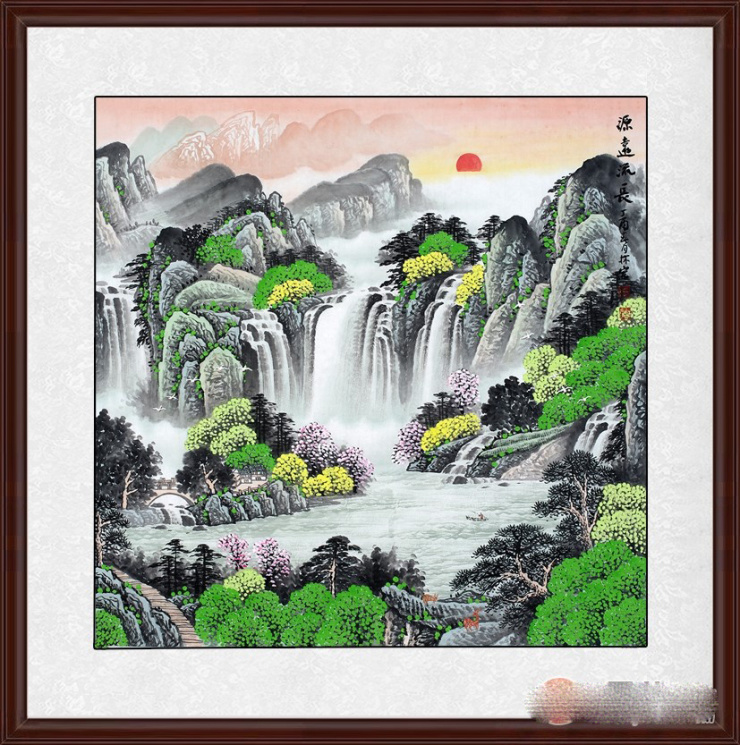都说开门见喜，喜从何来呢?那么春景怡人的景色绝对是长期雾霾中的人们最喜欢的景色，那么李林宏的这幅《源远流长》给人最具生机的感觉就是极佳的选择了，满眼绿色一种生命力的象征，小桥流水人家，桃源诗意也就是如此吧，下方的流水汇聚为一潭，有聚宝盆的聚财之意，青山秀水人杰灵，山水常在，杰灵常在，山水画让你的玄关熠熠生辉，财运滚滚!小鹿悠闲的栖息在这自然山水之中，显得十分的惬意，生活在喧嚣的闹市之中，一直渴望心中有着像桃园一般的净土环境，这样的山水画挂在玄关有韵味!更多玄关风水画选择尽在易从网

好消息!!!2017年【易从网】定制服务升级啦!

1、来图即可定制

假如我们商城没有您喜欢的山水画构图，您只需把高清图片发给客服，我们会安排签约的知名画家为您“一对一”创作，比如以“桃源山水画”而著称的画家石开、以“长城山水画”而著称的画家李林宏、以“青绿山水画”而著称的画家吴大恺等。

2、作品落款定制

若您看上了我们网站的山水画，却因个人爱好或者场合需要，想换个作品名字或者想加添几个字，比如领导升职时，您准备购买李林宏老师的长城山水画《中华之魂》当做礼物，可定制落款成《步步高升》，并加上自己的名字。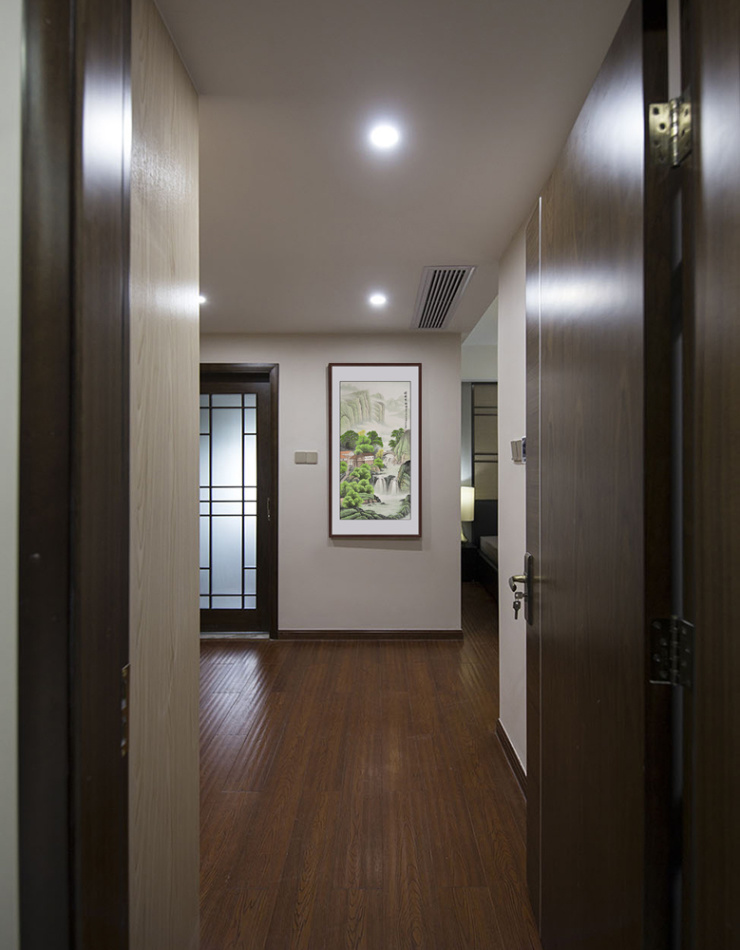——客户玄关李林宏《祥瑞聚福》山水画实拍

3、规格尺寸定制

比如您家客厅比较大，装修时也是专门定制的长沙发，不用担心，我们的画家从斗方小尺到五米巨幅山水画都有，毕竟山水画大的才更气派，您将所需的作品尺寸发给在线客服，便可以为您预约老师定制。

【易从网】，一家致力于传播中国传统书画文化，引领当代书画艺术品运用于装饰、送礼、收藏的专业字画商城。“让艺术走近大众，提升国民艺术水平”是我们的使命和追求。

`声明：本文由入驻焦点开放平台的作者撰写，除焦点官方账号外，观点仅代表作者本人，不代表焦点立场错误信息举报电话： 400-099-0099，邮箱：jubao@vip.sohu.com，或点此进行意见反馈，或点此进行举报投诉。`A B C D E F G H J K L M N P Q R S T W X Y Z
A - B - C - D - E
• A
• 鞍山
• 安庆
• 安阳
• 安顺
• 安康
• 澳门
• B
• 北京
• 保定
• 包头
• 巴彦淖尔
• 本溪
• 蚌埠
• 亳州
• 滨州
• 北海
• 百色
• 巴中
• 毕节
• 保山
• 宝鸡
• 白银
• 巴州
• C
• 承德
• 沧州
• 长治
• 赤峰
• 朝阳
• 长春
• 常州
• 滁州
• 池州
• 长沙
• 常德
• 郴州
• 潮州
• 崇左
• 重庆
• 成都
• 楚雄
• 昌都
• 慈溪
• 常熟
• D
• 大同
• 大连
• 丹东
• 大庆
• 东营
• 德州
• 东莞
• 德阳
• 达州
• 大理
• 德宏
• 定西
• 儋州
• 东平
• E
• 鄂尔多斯
• 鄂州
• 恩施
F - G - H - I - J
• F
• 抚顺
• 阜新
• 阜阳
• 福州
• 抚州
• 佛山
• 防城港
• G
• 赣州
• 广州
• 桂林
• 贵港
• 广元
• 广安
• 贵阳
• 固原
• H
• 邯郸
• 衡水
• 呼和浩特
• 呼伦贝尔
• 葫芦岛
• 哈尔滨
• 黑河
• 淮安
• 杭州
• 湖州
• 合肥
• 淮南
• 淮北
• 黄山
• 菏泽
• 鹤壁
• 黄石
• 黄冈
• 衡阳
• 怀化
• 惠州
• 河源
• 贺州
• 河池
• 海口
• 红河
• 汉中
• 海东
• 怀来
• I
• J
• 晋中
• 锦州
• 吉林
• 鸡西
• 佳木斯
• 嘉兴
• 金华
• 景德镇
• 九江
• 吉安
• 济南
• 济宁
• 焦作
• 荆门
• 荆州
• 江门
• 揭阳
• 金昌
• 酒泉
• 嘉峪关
K - L - M - N - P
• K
• 开封
• 昆明
• 昆山
• L
• 廊坊
• 临汾
• 辽阳
• 连云港
• 丽水
• 六安
• 龙岩
• 莱芜
• 临沂
• 聊城
• 洛阳
• 漯河
• 娄底
• 柳州
• 来宾
• 泸州
• 乐山
• 六盘水
• 丽江
• 临沧
• 拉萨
• 林芝
• 兰州
• 陇南
• M
• 牡丹江
• 马鞍山
• 茂名
• 梅州
• 绵阳
• 眉山
• N
• 南京
• 南通
• 宁波
• 南平
• 宁德
• 南昌
• 南阳
• 南宁
• 内江
• 南充
• P
• 盘锦
• 莆田
• 平顶山
• 濮阳
• 攀枝花
• 普洱
• 平凉
Q - R - S - T - W
• Q
• 秦皇岛
• 齐齐哈尔
• 衢州
• 泉州
• 青岛
• 清远
• 钦州
• 黔南
• 曲靖
• 庆阳
• R
• 日照
• 日喀则
• S
• 石家庄
• 沈阳
• 双鸭山
• 绥化
• 上海
• 苏州
• 宿迁
• 绍兴
• 宿州
• 三明
• 上饶
• 三门峡
• 商丘
• 十堰
• 随州
• 邵阳
• 韶关
• 深圳
• 汕头
• 汕尾
• 三亚
• 三沙
• 遂宁
• 山南
• 商洛
• 石嘴山
• T
• 天津
• 唐山
• 太原
• 通辽
• 铁岭
• 泰州
• 台州
• 铜陵
• 泰安
• 铜仁
• 铜川
• 天水
• 天门
• W
• 乌海
• 乌兰察布
• 无锡
• 温州
• 芜湖
• 潍坊
• 威海
• 武汉
• 梧州
• 渭南
• 武威
• 吴忠
• 乌鲁木齐
X - Y - Z
• X
• 邢台
• 徐州
• 宣城
• 厦门
• 新乡
• 许昌
• 信阳
• 襄阳
• 孝感
• 咸宁
• 湘潭
• 湘西
• 西双版纳
• 西安
• 咸阳
• 西宁
• 仙桃
• 西昌
• Y
• 运城
• 营口
• 盐城
• 扬州
• 鹰潭
• 宜春
• 烟台
• 宜昌
• 岳阳
• 益阳
• 永州
• 阳江
• 云浮
• 玉林
• 宜宾
• 雅安
• 玉溪
• 延安
• 榆林
• 银川
• Z
• 张家口
• 镇江
• 舟山
• 漳州
• 淄博
• 枣庄
• 郑州
• 周口
• 驻马店
• 株洲
• 张家界
• 珠海
• 湛江
• 肇庆
• 中山
• 自贡
• 资阳
• 遵义
• 昭通
• 张掖
• 中卫

1室1厅1厨1卫1阳台

1
2
3
4
5

0
1
2

1

1

0
1
2
3报名成功，资料已提交审核A B C D E F G H J K L M N P Q R S T W X Y Z
A - B - C - D - E
• A
• 鞍山
• 安庆
• 安阳
• 安顺
• 安康
• 澳门
• B
• 北京
• 保定
• 包头
• 巴彦淖尔
• 本溪
• 蚌埠
• 亳州
• 滨州
• 北海
• 百色
• 巴中
• 毕节
• 保山
• 宝鸡
• 白银
• 巴州
• C
• 承德
• 沧州
• 长治
• 赤峰
• 朝阳
• 长春
• 常州
• 滁州
• 池州
• 长沙
• 常德
• 郴州
• 潮州
• 崇左
• 重庆
• 成都
• 楚雄
• 昌都
• 慈溪
• 常熟
• D
• 大同
• 大连
• 丹东
• 大庆
• 东营
• 德州
• 东莞
• 德阳
• 达州
• 大理
• 德宏
• 定西
• 儋州
• 东平
• E
• 鄂尔多斯
• 鄂州
• 恩施
F - G - H - I - J
• F
• 抚顺
• 阜新
• 阜阳
• 福州
• 抚州
• 佛山
• 防城港
• G
• 赣州
• 广州
• 桂林
• 贵港
• 广元
• 广安
• 贵阳
• 固原
• H
• 邯郸
• 衡水
• 呼和浩特
• 呼伦贝尔
• 葫芦岛
• 哈尔滨
• 黑河
• 淮安
• 杭州
• 湖州
• 合肥
• 淮南
• 淮北
• 黄山
• 菏泽
• 鹤壁
• 黄石
• 黄冈
• 衡阳
• 怀化
• 惠州
• 河源
• 贺州
• 河池
• 海口
• 红河
• 汉中
• 海东
• 怀来
• I
• J
• 晋中
• 锦州
• 吉林
• 鸡西
• 佳木斯
• 嘉兴
• 金华
• 景德镇
• 九江
• 吉安
• 济南
• 济宁
• 焦作
• 荆门
• 荆州
• 江门
• 揭阳
• 金昌
• 酒泉
• 嘉峪关
K - L - M - N - P
• K
• 开封
• 昆明
• 昆山
• L
• 廊坊
• 临汾
• 辽阳
• 连云港
• 丽水
• 六安
• 龙岩
• 莱芜
• 临沂
• 聊城
• 洛阳
• 漯河
• 娄底
• 柳州
• 来宾
• 泸州
• 乐山
• 六盘水
• 丽江
• 临沧
• 拉萨
• 林芝
• 兰州
• 陇南
• M
• 牡丹江
• 马鞍山
• 茂名
• 梅州
• 绵阳
• 眉山
• N
• 南京
• 南通
• 宁波
• 南平
• 宁德
• 南昌
• 南阳
• 南宁
• 内江
• 南充
• P
• 盘锦
• 莆田
• 平顶山
• 濮阳
• 攀枝花
• 普洱
• 平凉
Q - R - S - T - W
• Q
• 秦皇岛
• 齐齐哈尔
• 衢州
• 泉州
• 青岛
• 清远
• 钦州
• 黔南
• 曲靖
• 庆阳
• R
• 日照
• 日喀则
• S
• 石家庄
• 沈阳
• 双鸭山
• 绥化
• 上海
• 苏州
• 宿迁
• 绍兴
• 宿州
• 三明
• 上饶
• 三门峡
• 商丘
• 十堰
• 随州
• 邵阳
• 韶关
• 深圳
• 汕头
• 汕尾
• 三亚
• 三沙
• 遂宁
• 山南
• 商洛
• 石嘴山
• T
• 天津
• 唐山
• 太原
• 通辽
• 铁岭
• 泰州
• 台州
• 铜陵
• 泰安
• 铜仁
• 铜川
• 天水
• 天门
• W
• 乌海
• 乌兰察布
• 无锡
• 温州
• 芜湖
• 潍坊
• 威海
• 武汉
• 梧州
• 渭南
• 武威
• 吴忠
• 乌鲁木齐
X - Y - Z
• X
• 邢台
• 徐州
• 宣城
• 厦门
• 新乡
• 许昌
• 信阳
• 襄阳
• 孝感
• 咸宁
• 湘潭
• 湘西
• 西双版纳
• 西安
• 咸阳
• 西宁
• 仙桃
• 西昌
• Y
• 运城
• 营口
• 盐城
• 扬州
• 鹰潭
• 宜春
• 烟台
• 宜昌
• 岳阳
• 益阳
• 永州
• 阳江
• 云浮
• 玉林
• 宜宾
• 雅安
• 玉溪
• 延安
• 榆林
• 银川
• Z
• 张家口
• 镇江
• 舟山
• 漳州
• 淄博
• 枣庄
• 郑州
• 周口
• 驻马店
• 株洲
• 张家界
• 珠海
• 湛江
• 肇庆
• 中山
• 自贡
• 资阳
• 遵义
• 昭通
• 张掖
• 中卫• 手机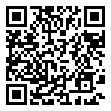• 分享
• 设计
免费设计
• 计算器
装修计算器
• 入驻
合作入驻
• 联系
联系我们
• 置顶
返回顶部